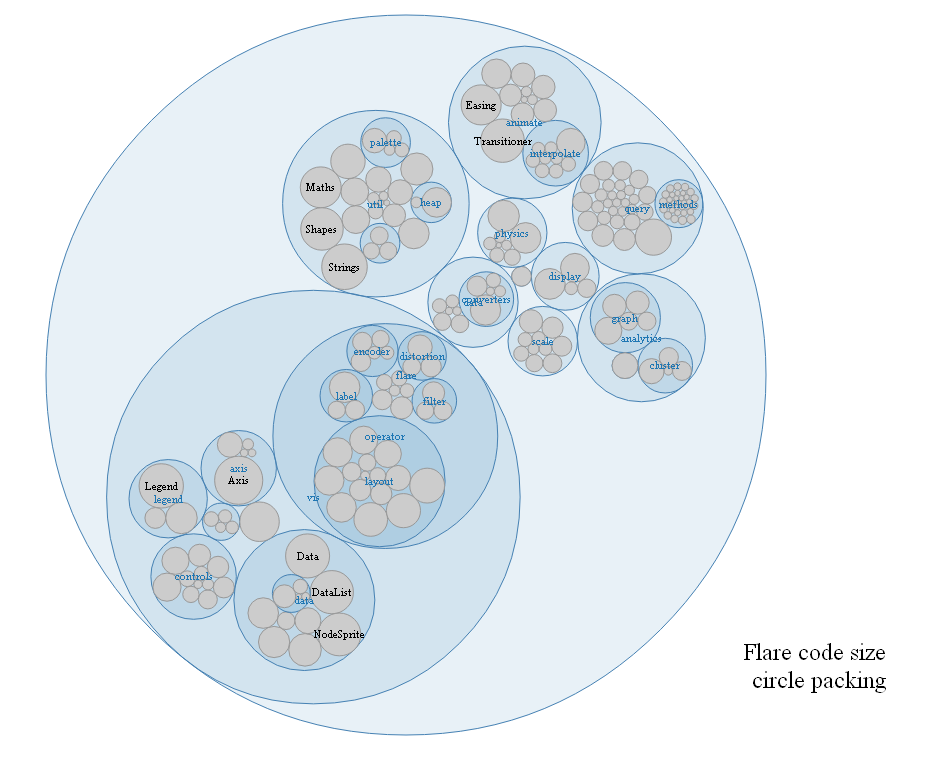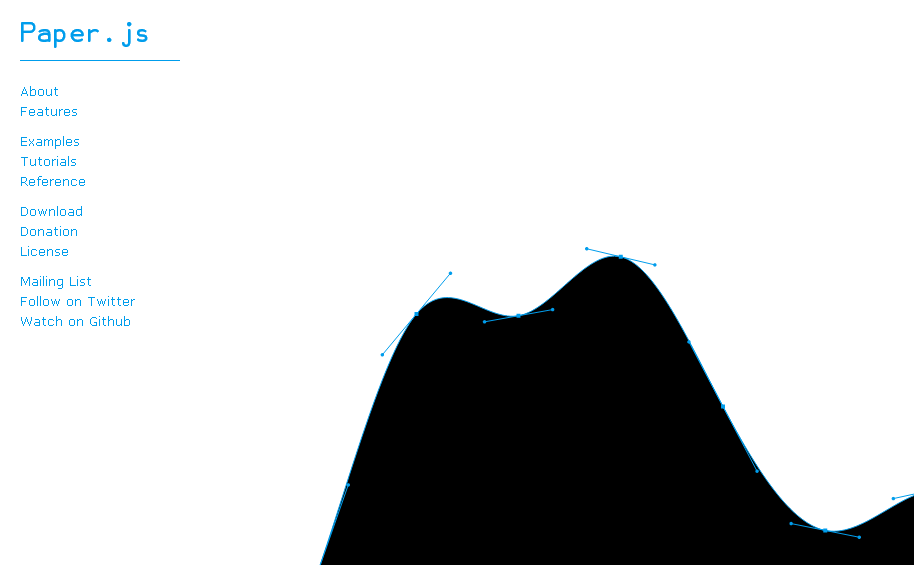# September 2012

You are currently browsing the monthly archive for September 2012.

## “Knowledge-Based General Game Playing”

In “Knowledge-Based General Game Playing”  Haufe, Michulke, Schiffel, and Thielscher (2011) discuss their general game playing program FLUXPLAYER.  They summarize their article as

“This article gives an overview of ﬁve essential methods for knowledge-based General Game Playing:
1. We show how to automatically generate evaluation functions for non-terminal positions.
2. We demonstrate the application of machine learning
techniques to improve these evaluation functions.
3. We illustrate how a system can automatically derive new
properties of games using automated theorem proving.
4. We present two specific techniques that help to reduce the
complexity of the search space by mimicking the ability
of humans to
(a) detect symmetries in games and
(b) identify subgames in composite games.”

FLUXPLAYER creates a fuzzy logic position evaluation function based on the rules for “for terminal and goal states”, persistent makers (such as pieces), a “logic-to-neural-network transformation”, simulations, temporal difference learning, symmetry detection, factoring (“Given its mere rules, how can a previously unknown game be automatically decomposed into independent subgames?”), automated theorem proving, and the $C- IL^2P$ algorithm.

## “Future impact: Predicting scientific success”

In “Future impact: Predicting scientific success“, Acuna, Allesina, and Kording predict the future h-index of scientist using their current h-index, the square root of the number of articles published, years since first publication, number of distinct journals, and the number of articles in top journals.  They vary the coefficients of a linear regression with the number of years in the forecast and note that, in the short term the largest coefficient is (not surprisingly) the scientist’s current h-index, but in the long term, the number of articles in top journals and the number of distinct journals become more important for the 10 year h-index forecast.  They achieve an $R^2$ value of 0.67 for neuro-scientists which is significantly larger than the $R^2$ using h-index alone (near 0.4).

Additionally, they provide an on-line tool you can use to make your own predictions.

## Data-Driven DocumentsAnother cool link from Carl.  “D3.js is a JavaScript library for manipulating documents based on data. “

## Paper.jsCarl sent me this link.  Check it out.  Fun!

## “Finite-time Analysis of the Multiarmed Bandit Problem”

In the paper “Finite-time Analysis of the Multiarmed Bandit Problem“, Auer, Cesa-Bianchi, and Fisher (2002) prove that for the fixed reward distributions, “the optimal logarithmic regret is also achievable uniformly over time, with simple and efficient policies, and for all reward distributions with bounded support.”

The paper discusses the following three ideas:

1) The expected regret for the upper confidence bound algorithm UCB1 is bounded above by

$$\left[ 8 \sum_{i:\mu_i < \mu^*} \left({\log n \over {\mu^* - \mu_i}}\right)\right] + \left( 1 + {\pi^2\over 3}\right)\left(\sum_{j=1}^K (\mu^*-\mu_j) \right)$$

where $\mu_i$ is the mean of the $i$th distribution, $n$ is the number of pulls, and $K$ is the number of arms if the rewards are between 0 and 1.

2)  They improve on the coefficient of $\log n$ with a slightly more complex algorithm UCB2.

3)  The $\epsilon_n = 1/n$ greedy algorithm has $O(\log n)$ regret.

They conclude the paper with numerical results.

## Sleeping Experts

In “Regret Bounds for Sleeping Experts and Bandits”  Kleinberg, Niculescu-Mizil, and Sharma (2008) propose an algorithm for selecting among a list of experts some of whom may be sleeping.  At each time step $t$, the algorithm ranks the experts in order of preference with a permutation vector $\sigma(t)$.   The first analyzed algorithm is Follow The Awake Leader (FTAL) for the case where all of the expert predictions and rewards are observed.  Next they explore a natural extension of upper confidence bound algorithm for the multi-armed bandit setting.  In the adversarial setting, they prove that the regret is at least $O(\sqrt{t\, K \log K})$ for $K$ bandits at time $t$.

## “Mixture of Vector Experts”

Mixture of Vector Expertsby HendersonShawe-Taylor, and Zerovnik (2005) describes an algorithm for mixing the predictions of several experts. Each expert gives at prediction vector in $\{0,1\}^n$ at time $t$.  Their experimental evidence suggests that their algorithm outperforms a similar componentwise scalar mixture of experts.

## Gambling in a rigged casino

In “Gambling in a rigged casino: The adversarial multi-armed bandit problem” Auer, Cesa-Bianchi, Freund, and Schapire (1998) give an algorithm for adversarial multi-armed bandits with at worst $O(\sqrt{T K \log K })$ regret for $K$ bandits over $T$ tries which is similar to the weighted majority algorithm. They also establish a lower bound on the regret of $O(\sqrt{T K})$ for the adversarial case.  In section 9, the authors prove that this algorithm can be applied to two person finite games to compute the value of the game for both players.

The paper develops four algorithms in sequence called Hedge, Exp3, Exp3.1, and Exp4.   Each algorithm uses a previous algorithm as a subroutine.  Hedge is a full information algorithm that requires observation of the untried arms after choosing one arm.  Exp3 only requires a bound on the maximum expected value possible at time $T$ and Exp3.1 operates by guessing the maximal expected value.  Exp4 is a mixture of experts algorithm.

In the related paper “The non-stochastic multi-armed bandit problem” by the same authors, the algorithms are extended and the regret bounds are improved. At the end of this paper, they explore applications to Game Theory.

## Using Bregman Distance to Grow Decision Trees

In “Statistical Learning Algorithms Based on Bregman Distances“,  La erty, Pietra, and Pietra (1999) take a fairly standard entropy based tree growing algorithm and replace KL distance with Bregman distance. “In the feature selection step, each linear constraint in a pool of candidate features is evaluated by the reduction in Bregman distance that would result from adding it to the model.”  This is reminiscent of the distribution update step in Softboost and the “information projection” view of Adaboost (See Boosting as Entropy Projection).  The paper is somewhat technical containing interesting theorem proving techniques.

## Poll Results: Top Analytics, Data Mining, Big Data software used

Kdnuggets.com posted these poll results on data mining software.  Looks like R, Excel, Rapid-I RapidMiner, KNIME, and Weka/Pentaho were the most popular.